Q-Chem 5.0 User’s Manual

# 11.7 Frozen-Density Embedding Theory based methods

Frozen-Density Embedding Theory (FDET) [498, 499] provides a formal framework in which the whole system is described by means of two independent quantities: the embedded wave function (interacting or not) and the density associated with the environment. The total energy equation in frozen density embedding theory for a wave function in state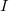embedded in a environment density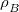reads (for definitions see Table 11.7):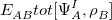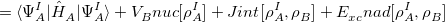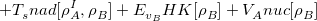(11.86)

The embedding operator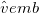, which is added to the Hamiltonian of subsystem A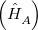, is given in the form of a potential: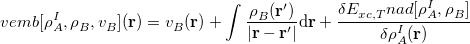(11.87)

The last term (non-electrostatic component) in equation  causes the embedding potential to be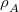-dependent, which in return induces an inconsistency between the potential and the energy. In the canonical form of FDET (conventional FDET) this inconsistency is addressed by performing macrocycles in which the embedding potential is repeatedly constructed using the current (embedded) density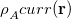after each cycle until self-consistency is reached.

In linearized FDET the non-additive energy functionals (for abbreviation denoted as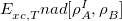) are each approximated by a functional which is linear in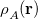. The approximation is constructed as a Taylor expansion of the non-additive energy functional at a reference density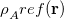with the series being truncated after the linear term.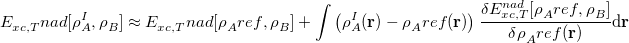(11.88)

In contrast to conventional FDET, the embedding potential then becomes-independent and macrocycles are no longer necessary. Another consequence of the linearization is that orthogonality between states is maintained since the same potential is used for all states.

## 11.7.1 FDE-ADC

FDE-ADC  is a density embedding method based on the combination of the Algebraic Diagrammatic Construction scheme for the polarization propagator (ADC, Section 6.8) and Frozen-Density Embedding Theory (FDET). In this particular variant the subsystem A is represented by a wave function whereas subsystem B is described by a density. The FDE-ADC method uses the linearized FDET approximation .

### 11.7.1.1 FDE-ADC Job Control

The FDE-ADC job control is accomplished in two sections, $rem and$fde. Enabling FDE-ADC, specification of the ADC method and other ADC job control parameters (thresholds, max. iterations etc) should be set in the $rem section. FDE-ADC also supports the excited state analysis (STATE_ANALYSIS) carried out by the libwfa module. The fragments are specified via the fragment descriptors (see Section 12) in the$molecule section, whereas the first fragment corresponds to the embedded species (A) while the second fragment represents the environment (B).

Note: The current implementation allows only for closed shell fragments.

FDE
 Turns density embedding on.

TYPE:
 BOOLEAN

DEFAULT:
 False

OPTIONS:
 True Perform an FDE-ADC calculation. False Don’t perform FDE-ADC calculation.

RECOMMENDATION:
 Set the $rem variable FDE to TRUE to start a FDE-ADC calculation. METHOD  Determines which FDE-ADC method should be used if FDE = True. TYPE:  STRING DEFAULT:  None OPTIONS:  adc(2) Perform an FDE-ADC(2)-s calculation. adc(2)-x Perform an FDE-ADC(2)-x calculation. adc(3) Perform an FDE-ADC(3) calculation (potential constructed with MP(2) density). cvs-adc(2) Perform an FDE-ADC(2)-s calculation of core excitations. cvs-adc(2)-x Perform an FDE-ADC(2)-x calculation of core excitations. cvs-adc(3) Perform an FDE-ADC(3) calculation of core excitations. RECOMMENDATION:  None The FDE-ADC job control with respect to embedding parameters is accomplished via options in the$fde input section. The format of the $fde section requires key and value pairs separated by a space character: $fde
<Keyword>  <parameter>
$end  Note: The following job control variables belong only in the$fde section. Do not place them in the $rem section. The super-molecular expansion (SE) uses the full basis set of the super-system for calculations on each fragment. Because of the computational cost this option should only be used for small to medium sized super-systems. Note that for visualization of orbitals or densities SE only supports the generation of volumetric data via MAKE_CUBE_FILES (MolDen files are not supported, i.e. MOLDEN_FORMAT should be avoided). The reassembling of density matrix (RADM)  option allows for calculations on larger systems by only including the basis functions of the embedded species for the ADC calculation. RADM introduces an approximation for the construction of the embedding potential by using an artificially (but cheaply) constructed density matrix for subsystem A. With RADM, all regular options for visualization are supported (MAKE_CUBE_FILES and MOLDEN_FORMAT). The RADM option is the recommended choice for an FDE-ADC calculation. Analogous to a regular DFT calculation in Q-Chem(by using METHOD) the exchange-correlation functional combination can either be selected with one keyword XC_Func,or by defining X_Func and C_Func (similar to EXCHANGE and CORRELATION). T_Func  Kinetic energy functional used for the construction of the embedding potential. INPUT SECTION:$fde
TYPE:
 STRING

DEFAULT:
 None

OPTIONS:
 TF Use Thomas-Fermi kinetic energy functional.

RECOMMENDATION:
 None

XC_Func
 Exchange-Correlation functional used for the construction of the embedding potential.

INPUT SECTION: $fde TYPE:  STRING DEFAULT:  None OPTIONS:  All LDA/GGA exchange-correlation functionals available in Q-Chem. RECOMMENDATION:  Only use LDA or GGA-type functionals. X_Func  Exchange functional used for the construction of the embedding potential. INPUT SECTION:$fde
TYPE:
 STRING

DEFAULT:
 None

OPTIONS:
 All LDA/GGA exchange functionals available in Q-Chem.

RECOMMENDATION:
 Only use LDA or GGA-type functionals. XC_Func and X_Func are mutually exclusive.

C_Func
 Exchange-Correlation functional used for the construction of the embedding potential.

INPUT SECTION: $fde TYPE:  STRING DEFAULT:  None OPTIONS:  All LDA/GGA correlation functionals available in Q-Chem. RECOMMENDATION:  Only use LDA or GGA-type functionals. XC_Func and C_Func are mutually exclusive. Expansion  Specifies which basis set expansion should be used. INPUT SECTION:$fde
TYPE:
 STRING

DEFAULT:
 None

OPTIONS:
 SE/super/supermolecular Supermolecular basis is used for both System A and B. RADM Use RADM approximation (see above).

RECOMMENDATION:
 SE should be used for testing purposes only since it is very expensive for large systems. Use the RADM approximation for larger systems.

rhoB_method
 Method to calculate the environment density (B).

INPUT SECTION: $fde TYPE:  STRING DEFAULT:  None OPTIONS:  HF Use Hartree-Fock method. DFT Use Density Functional Theory. RECOMMENDATION:  If DFT is specified, the respective exchange-correlation functional has to defined using the keyword XC_FUNC_B or X_FUNC_B and C_FUNC_B. XC_Func_B  Exchange-Correlation functional used for the environment DFT calculation. INPUT SECTION:$fde
TYPE:
 STRING

DEFAULT:
 None

OPTIONS:
 All LDA/GGA/global-hybrid-GGA exchange-correlation functionals available in Q-Chem.

RECOMMENDATION:
 None

X_Func_B
 Exchange functional used for the environment DFT calculation.

INPUT SECTION: $fde TYPE:  STRING DEFAULT:  None OPTIONS:  All LDA/GGA exchange functionals available in Q-Chem. RECOMMENDATION:  XC_Func_B and X_Func_B are mutually exclusive. C_Func_B  Correlation functional used for the environment DFT calculation. INPUT SECTION:$fde
TYPE:
 STRING

DEFAULT:
 None

OPTIONS:
 All LDA/GGA correlation functionals available in Q-Chem.

RECOMMENDATION:
 XC_Func_B and C_Func_B are mutually exclusive.

PrintLevel
 Print level for FDE-ADC output.

INPUT SECTION: $fde TYPE:  INTEGER DEFAULT:  0 OPTIONS:  0 minimum print level 1 extended print level 2 maximum print level 3 max. print level and additional text files (densities, etc.) RECOMMENDATION:  None ### 11.7.1.2 Examples Example 11.297 Input for a FDE-ADC(2)/cc-pVDZ calculation in supermolecular expansion on CO embedded in one water molecule. $rem
SYM_IGNORE           = true
METHOD               = adc(2)
EE_STATES            = 2
BASIS                = cc-pvdz
FDE                  = true
MEM_STATIC           = 1024
MEM_TOTAL            = 16000
ADC_DAVIDSON_MAXITER = 100
ADC_DAVIDSON_CONV    = 5
$end$molecule
0 1
--
0 1
C    -3.618090    1.376803   -0.020795
O    -4.735683    1.525556    0.115023
--
0 1
O    -7.956372    1.485406    0.116792
H    -6.992316    1.421133    0.177470
H    -8.105846    2.442220    0.111599
$end$fde
T_Func       TF
XC_Func      PBE
expansion    super
rhoB_method  HF
\$end


### 11.7.1.3 FDE-ADC output

In general the FDE-ADC output indicates all important stages of the FDE-ADC calculation, which are:

1. Generation of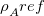,

2. Generation of,

3. Construction of the embedding potential,

4. Start of FDE-ADC calculation and

5. Final FDE-ADC summary.

In the following table definitions of the terms printed to the output are collected. These quantities are printed for every state, i.e. for every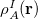. In addition, the non-electrostatic interactions with respect to the reference densityare printed at the top of the FDE-ADC summary.

 Subsystem Energies Embedded system (A)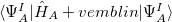Environment (B)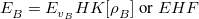Electrostatic Interactions rho_A <->  rho_B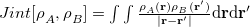rho_A <->  Nuc_B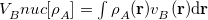rho_B <->  Nuc_A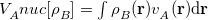Nuc_A <->  Nuc_B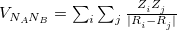Non-Electrostatic Interactions non-additive E_xc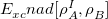non-additive T_s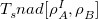integrated v_xc nad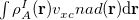integrated v_T nad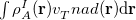Final FDE-ADC energies Delta_Lin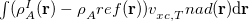Final Energy (A)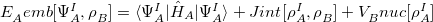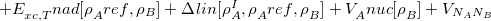Final Energy (A+B)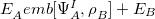Table 11.7: Definition of output terms.